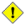## Name

= — 如果几何/地理 A 的坐标和坐标顺序与几何/地理 B 的坐标和坐标顺序相同，则返回` TRUE`

## Synopsis

`boolean =(` geometry A , geometry B `)`;

`boolean =(` geography A , geography B `)`;

## 描述只有在所有方面都完全相同、具有相同坐标、相同顺序的几何/地理才被该运算符视为相等。 对于“空间平等”，它忽略坐标顺序等内容，并且可以检测以不同表示覆盖相同空间区域的要素，请使用ST_OrderingEquals 或 ST_Equals此运算符不对几何图形使用任何有效索引。 要使用索引执行稳健的相等性测试，请使用= —和 &&。此方法支持圆形字符串和曲线。该函数支持多面体曲面。

## 示例

```SELECT 'LINESTRING(0 0, 0 1, 1 0)'::geometry = 'LINESTRING(1 1, 0 0)'::geometry;
?column?
----------
f
(1 row)

SELECT ST_AsText(column1)
FROM ( VALUES
('LINESTRING(0 0, 1 1)'::geometry),
('LINESTRING(1 1, 0 0)'::geometry)) AS foo;
st_astext
---------------------
LINESTRING(0 0,1 1)
LINESTRING(1 1,0 0)
(2 rows)

-- Note: the GROUP BY uses the "=" to compare for geometry equivalency.
SELECT ST_AsText(column1)
FROM ( VALUES
('LINESTRING(0 0, 1 1)'::geometry),
('LINESTRING(1 1, 0 0)'::geometry)) AS foo
GROUP BY column1;
st_astext
---------------------
LINESTRING(0 0,1 1)
LINESTRING(1 1,0 0)
(2 rows)

-- In versions prior to 2.0, this used to return true --
SELECT ST_GeomFromText('POINT(1707296.37 4820536.77)') =
ST_GeomFromText('POINT(1707296.27 4820536.87)') As pt_intersect;

--pt_intersect --
f

```